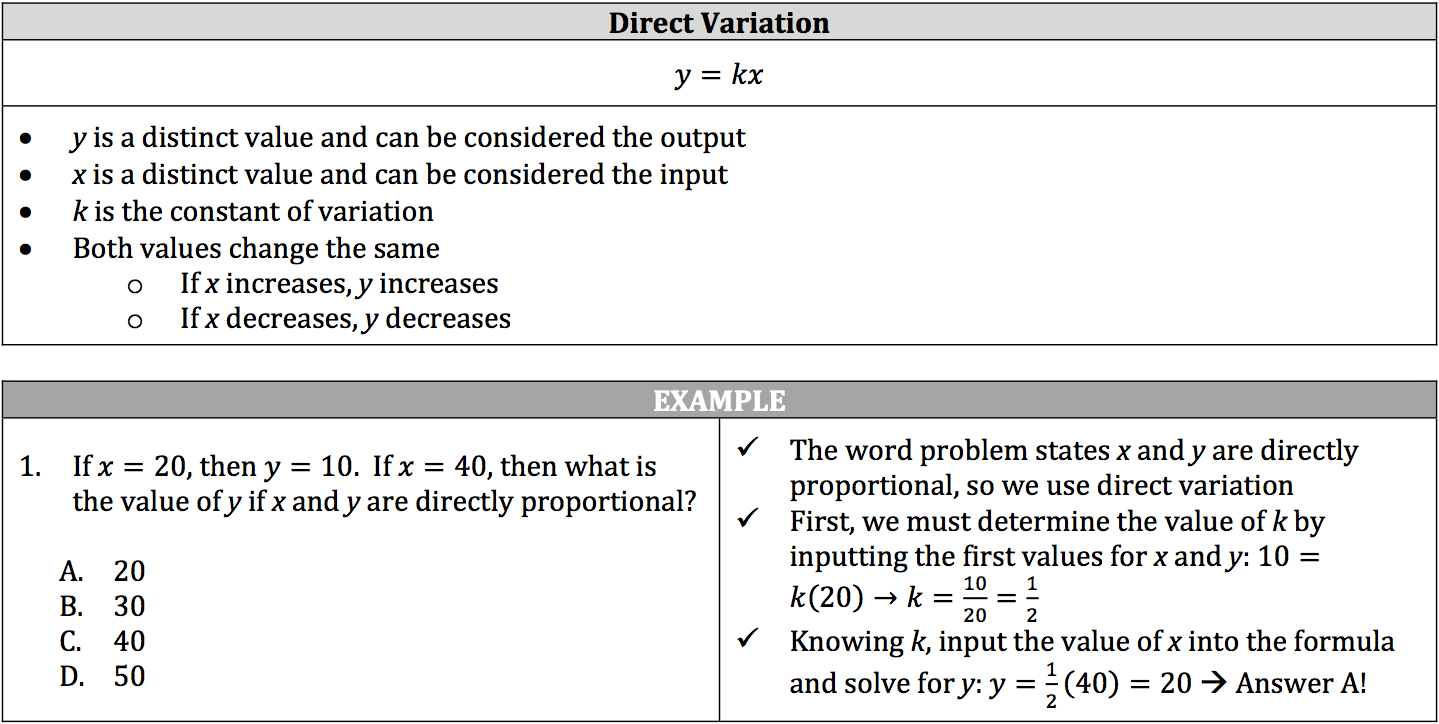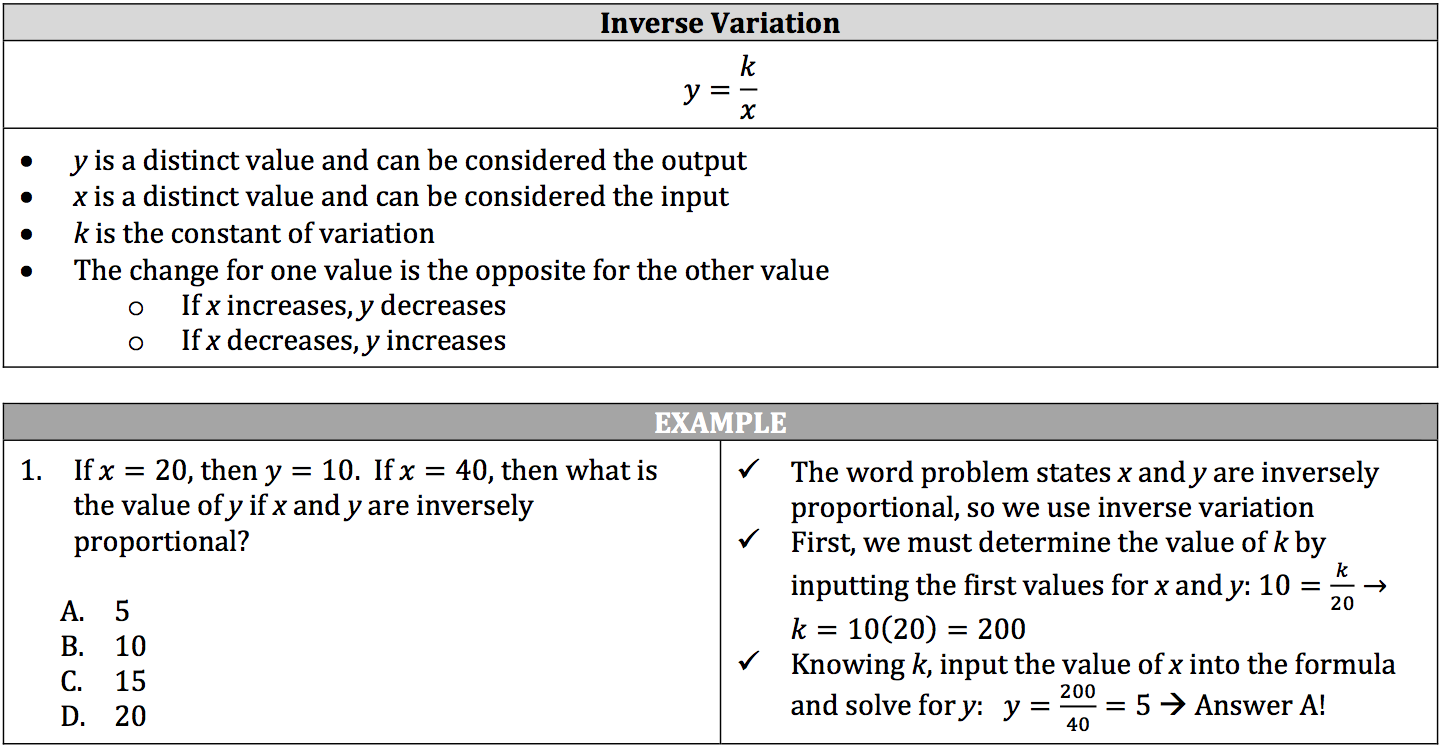Direct and inverse variation refers to two values that are proportional to one another depending on a particular constant, or k:

• Direct variation multiplies the constant to one of the values.
• Inverse variation divides the constant by one of the values.

To determine which variation to use, look closely at the words used in the question:

• If the question states that a value is directly proportional or varies directly with another value, then you use direct variation.
• If the question states that a value is inversely proportional or varies inversely with another value, then you use inverse variation.

### Using Direct Variation### Using Inverse Variation